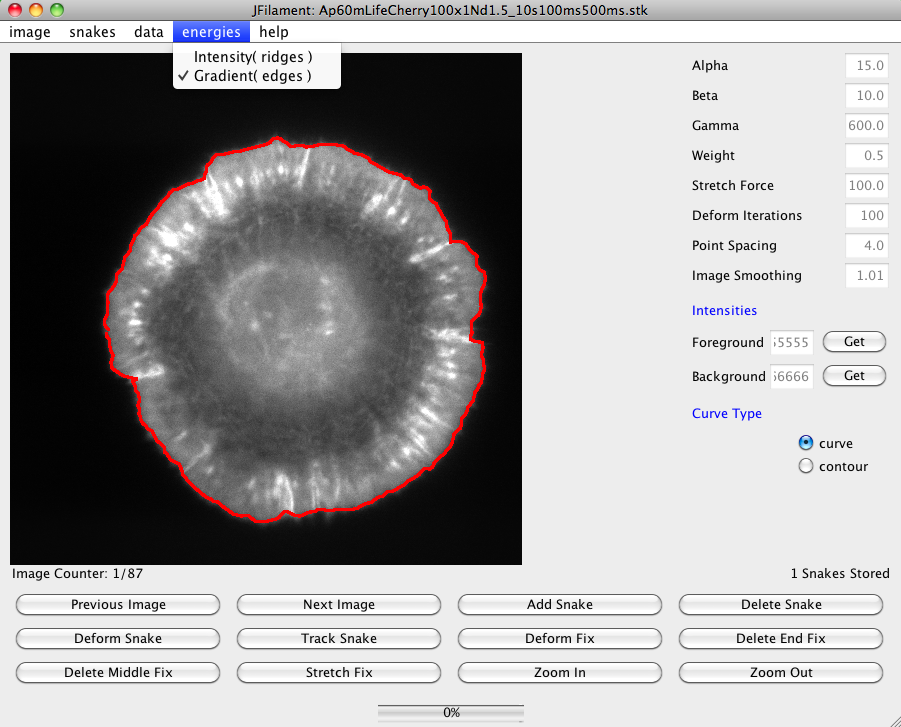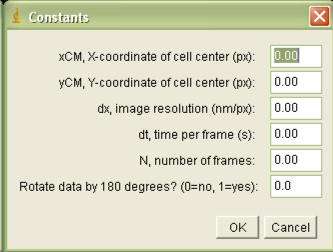# Leading Edge Position and Velocity Calculations

 JFilament Speckle TrackerJ Leading Edge Position and Velocity Leading Edge Intensity Correlation Analysis Speckle Velocities

LE_Velocity calculates the radial leading edge velocity of cells from active contours or "snakes."
JFilament must be used to fit snakes to the edge of cells using the "gradient" energy option (see below).Track snakes between time frames to generate a contour that evolves over time. Upon completion of tracking JFilament allows you to save a file containing points along the contour, "snakes.txt." This is the input required for leading edge edge position  calculation by the plugin

maps the active contours provided by the output of JFilament to polar coordinates. When LE_Radius is run it will prompt the user to input necessary data:The required parameters are:
•  xCM: the x-coordinate of the cell center, measured in pixels
• yCM: the y-coordinate of the cell center, measured in pixels
• dx: image resolution, measured in nm/pixel
• dt: time per frame, measured in s
• N: number of frames in the stack
• Rotation, which allows the user to rotate the angles of the ouput by 180 degrees. (Useful for collecting continuous ribbons of sections of cells.)
Once the user inputs these data, LE_Radius will prompt the user to choose a snake file.

Output data file: Contains a
Nx360 matrix of cell
radii. The column number of this matrix indicates the frame number, while the rows are different angles. The radius will be saved in units of microns.

The plugin LE_Velocity maps the active contours provided by the output of JFilament to polar coordinates. After this conversion, it calculates the radial velocity of the leading edge between subsequent frames as a function of angle around the cell and time. It requires that an image stack be open in ImageJ. When  LE_Velocity is run it will prompt the user to input necessary data:

The required parameters are:

•  xCM: the x-coordinate of the cell center, measured in pixels
• yCM: the y-coordinate of the cell center, measured in pixels
• dx: image resolution, measured in nm/pixel
• dt: time per frame, measured in s
• M: the number of time frames used for velocity calculations
• N: number of frames in the stack
• S: scaling factor for the velocity vector flow maps.
Once the user inputs these data, LE_Velocity will prompt the user to choose a snake file.

Output data file:
Contains the calculated velocity data in two formats. The first, a duplicate stack displaying vector flow maps of speckle velocities. A value of S=1 yields velocity vectors that scale 1nm/s per pixel. The second is a data file containing a Nx360 matrix of velocities. The column number of this matrix indicates the frame number, while the rows are different angles. The velocity will be saved in units of nm/s.

Note: For open active contours (such as for sections of cells) the velocities near the contour edge may fluctuate artificially because of contour end fluctuations.
If there are no active contour points at a given angle at a specific time, a velocity of 0 nm/s will be saved for that angle.

References:

• G. L. Ryan, N. Watanabe, D. Vavylonis, "Image Analysis Tools to Quantify Cell Shape and Protein Dynamics near the Leading Edge," Cell Structure and Function (2013).
• G. L. Ryan, H. Petroccia, N. Watanabe, D. Vavylonis, "Excitable Actin Dynamics in Lamellipodial Protrusion and Retraction," Biophys. J. 102:1493-1502 (2012).